﻿ 扭曲向列型液晶空间光调制器偏振分光特性的实验测试 Experimental Test for Polarized Splitting Light Characteristic of Twisted Nematic Liquid-Crystal Spatial Light Modulator

Instrumentation and Equipments
Vol.06 No.01(2018), Article ID:24079,8 pages
10.12677/IaE.2018.61003

Experimental Test for Polarized Splitting Light Characteristic of Twisted Nematic Liquid-Crystal Spatial Light Modulator

Binhu Wang, Yongxiang Xu, Fuzhong Bai*

School of Mechanical Engineering, Inner Mongolia University of Technology, Hohhot Inner Mongolia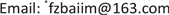Received: Feb. 28th, 2018; accepted: Mar. 12th, 2018; published: Mar. 19th, 2018ABSTRACT

When a linearly polarized light is incident to a twisted nematic liquid-crystal spatial light modulator (TN-LCSLM), the relationships of output intensity and rotating angles of two polarizers placed in front and behind of it are tested experimentally. Cooperated with theoretical analysis, it confirms the principle of polarized splitting light and composed intensity of TN-LCSLM with twisted angle of 90° under zero gray image unloaded. The studied conclusion can provide new idea for clearly understanding the TN-LCSLM’S modulation characteristic.

Keywords:Twisted Nematic Liquid-Crystal Spatial Light Modulator (TN-LCSLM), Crystal Birefringence, Polarized Splitting Light, Characteristic Test1. 引言

LCSLM按照控制信号不同可分为光寻址和电寻址两类；根据其调制对象的不同可分为振幅型、相位型、复合型；按照光输出方式分为反射式和透射式两种；按照液晶内部分子排列可分为扭曲相列型(Twisted Nematic LCSLM，简称TN-LCSLM)和零扭曲相列型(Zero Twisted Nematic LCSLM，简称ZTN-LCSLM)。TN-LCSLM也属于向列型液晶空间光调制器，不加电压时内部液晶分子从前表面到后表面呈递进扭曲，总扭曲角一般为90˚。TN-LCSLM具有旋光作用，可以对入射光束振幅、相位和偏振进行调制，是当代处理光学信息的重要器件。

2. 理论分析

2.1. 液晶双折射原理

TN-LCSLM液晶分子结构排列模型如图1所示。根据晶体的双折射效应    ，线偏振光束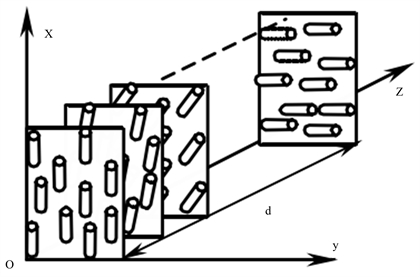Figure 1. Molecular structure model of TN-LCSLM

TN-LCSLM液晶分子的排列从前表面至后表面逐层递进发生扭曲，每一层上所有液晶分子的扭曲角相等。当液晶前后表面不施加电场时，液晶分子自然扭曲；当所加电场超过阈值电压后，液晶分子沿电场方向发生偏转。液晶片厚度为d，入射光波长为λ，o光和e光之间的相位差可表示为：

$\delta =\frac{2\text{π}}{\lambda }|{n}_{o}-{n}_{e}|d$ (1)

2.2. 光学实验结构

2.3. 偏振分光与强度合成原理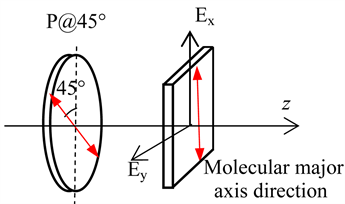Figure 2. Liquid crystal molecular birefraction sketch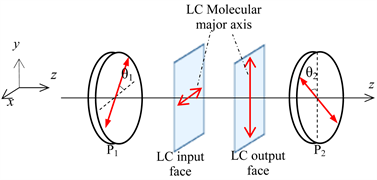Figure 3. Optical configuration of experimental test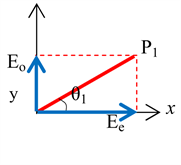(a)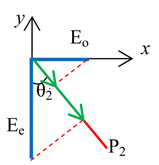(b)

Figure 4. Geometric vector diagram between polarizer P1 and input face of TN-LCSLM (a); polarizer P2 and output face (b)

$\begin{array}{l}{E}_{x}=A\mathrm{cos}{\theta }_{1}\\ {E}_{y}=A\mathrm{sin}{\theta }_{1}\end{array}$(2)

e光由x轴方向旋转至y轴方向(后表面液晶长轴方向)，并将引入相位调制δ，即

${{E}^{\prime }}_{y}=A\mathrm{cos}{\theta }_{1}{\text{e}}^{i\delta }$ (3)

o光由y轴方向旋转至x轴方向，复振幅不发生变化，即

${{E}^{\prime }}_{x}=A\mathrm{sin}{\theta }_{1}$ (4)

$\begin{array}{l}{{E}^{″}}_{y}=A\mathrm{cos}{\theta }_{1}\mathrm{cos}{\theta }_{2}{\text{e}}^{i\delta }\\ {{E}^{″}}_{x}=A\mathrm{sin}{\theta }_{1}\mathrm{sin}{\theta }_{2}\end{array}$ (5)

$I={|{{E}^{″}}_{x}+{{E}^{″}}_{y}|}^{2}={A}^{2}{\mathrm{cos}}^{2}\left({\theta }_{1}-{\theta }_{2}\right)-{A}^{2}\mathrm{sin}2{\theta }_{1}\mathrm{sin}2{\theta }_{2}{\mathrm{sin}}^{2}\left(\delta /2\right)$ (6)

$I={A}^{2}{\mathrm{cos}}^{2}\left(\frac{\text{π}}{4}-{\theta }_{2}\right)$ (7)

$I={A}^{2}{\mathrm{cos}}^{2}{\theta }_{1},\text{}{\theta }_{2}={0}^{\circ }$ (8)

$I={A}^{2}{\mathrm{cos}}^{2}\left({\theta }_{1}-\frac{\text{π}}{2}\right),\text{}{\theta }_{2}={90}^{\circ }$ (9)

3. 实验及结果分析

3.1. 偏振片P2旋转角度对出射光强的影响

3.2. 偏振片P1旋转角度对出射光强的影响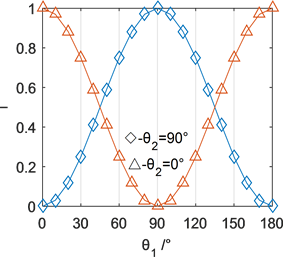(a)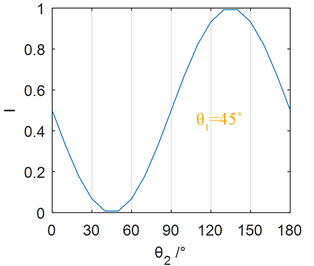(b)

Figure 5. Simulated curves of the relation between the exiting intensity and θ2 and θ1 respectively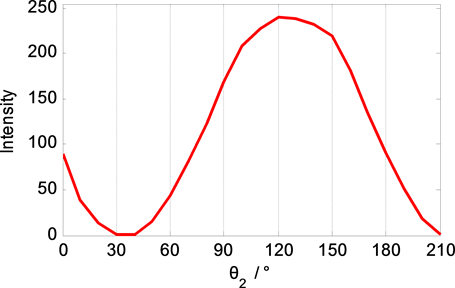Figure 6. Experimental result of exiting intensity vs. θ2 under θ1 = 45˚

140˚、以10˚为步长进行变化；共记录18个光强数据。另外，将偏振片P2旋转至θ2 = 90˚的位置，相当于出射光中仅包含o光分量，重复上述实验过程。两次测试得到的实验结果如图7所示。

1) 实验结果近似符合余弦曲线规律，并且变化趋势与仿真结果相近。

2) 当θ2 = 0˚时，偏振片P2的振动方向与e光振动方向平行，因此出射光中仅包含e光分量。此时出射强度的变化是由于旋转P1引起入射光在液晶分子长轴方向上的分量的变化而造成。当θ1接近0˚时，入射线偏振光的振动方向与液晶分子长轴方向平行，进入液晶的e光分量达到最大值，因此出射光强也达到最大值。

3) 当P1处于θ1 = −45˚、45˚和135˚这几个位置时，o光和e光的强度相等；这说明，此时线偏振光入射至液晶后产生两个振幅相等的偏振分量。进一步地，上述角度之间相差90˚、包括图7中两条曲线相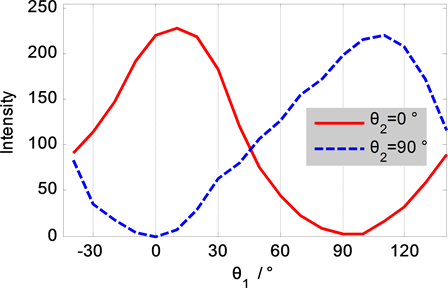Figure 7. Experimental result of exiting intensity vs. θ1

4. 结论

Experimental Test for Polarized Splitting Light Characteristic of Twisted Nematic Liquid-Crystal Spatial Light Modulator[J]. 仪器与设备, 2018, 06(01): 12-19. https://doi.org/10.12677/IaE.2018.61003

1. 1. 徐展斌, 应朝福, 林培秋, 等. 纯相位液晶空间光调制器相位调制特性的优化[J]. 浙江师范大学学报(自然科学版), 2009, 32(2): 164-168.

2. 2. 蔡冬梅, 薛丽霞, 凌宁, 等. 液晶空间光调制器相位调制特性研究[J]. 光电工程, 2007, 34(11): 19-23.

3. 3. Lu, K.H. and Saleh, B.E.A. (1990) Theory and Design of the Liquid Crystal TV as an Optical Spatial Phase Modultor. Optical Engineering, 29, 240-245. https://doi.org/10.1117/12.55584

4. 4. Cox, I.J. and Mille, M. (1997) A Review of Wa-termarking and Importance of Perceptual Modelling Human Vision and Imaging. Proceedings of SPIE, 20, 181-184.

5. 5. 马靖, 裴丽燕, 许灿华, 周雄图. 用偏光干涉法动态测量扭曲向列型液晶指向矢[J]. 光子学报, 2016, 45(12): 1226001.

6. 6. 李大海, 潭泽富, 陈怀新, 等. 采用环路径向剪切干涉法测量液晶电视的相位调制特性[J]. 光子学报, 2002, 31(8): 970-974.

7. 7. 苗悦, 白福忠, 刘珍, 田朝平, 梅秀庄. 采用共光路干涉法测量液晶空间光调制器的相位调制特性[J]. 激光与光电子学进展, 2016, 53(2): 129-134.

8. 8. Moreno, I., Davis, J.A., Klein, F.A., et al. (2008) Polarization-Splitting Common-Path Interferometer Based on a Zero Twist Liquid Crystal Display. Applied Optics, 47, 1797-1801. https://doi.org/10.1364/AO.47.001797

9. 9. Sarkadi, T. and Kop, P. (2011) Measurement of the Jones Matrix of Liquid Crystal Displays Using a Common Path Interferometer. Journal of Optics, 12, Article ID: 035404.

10. 10. Davis, J.A., Pascoguin, B.M.L., Moreno, I. and Nava-Veg, A. (2009) Circu-lar-Polarization-Splitting Common-Path Interferometer Based on a Zero-Twist Liquid-Crystal Display. Optics Letters, 34, 1486-1488. https://doi.org/10.1364/OL.34.001486

11. 11. 刘伯晗, 吴丽莹, 张健. 一种用于衍射光元件优化设计的快速算法的研究[J]. 光学学报, 2007, 27(2): 219-224.

12. 12. Li, B.-X., Borshch, V., Shiyanovskii, S.V., Liu, S.-B. and Lavrentovich, O.D. (2014) Electro-Optics Switching of Dielectrically Negative Nematic through Nanosecond Electric Modification of Order Parameter. Applied Physics Letters, 104, 201105. https://doi.org/10.1063/1.4879018

13. 13. 余湛. 基于液晶空间光调制器光束整形的理论算法和实验研究[D]: [硕士学位论文]. 长沙: 国防科学技术大学, 2010.

14. 14. Leger, J.R., et al. (1998) Coherent Laser Beam Addition: An Application of Binary Optics Technology. Lincoln Laboratory Journal, 1, 255-246.

15. 15. Liang, J.Y., Kohn, R.N., Becker, M.F., et al. (2009) 1.5% Root-Mean-Square Flat-Intensity Laser Beam Formed Using a Binary-Amplitude Spatial Light Modulator. Applied Optics, 48, 1955-1968. https://doi.org/10.1364/AO.48.001955

16. 16. Bai, F.Z., Miao, Y., Xu, Y.X., Wang, G. and An, P. (2017) Absolute Measure-ment of the Generated Aberrations from Liquid Crystal Spatial Light Modulator Using a Common-Path Interferometry. Journal of Modern Optics, 64, 1257-1262. https://doi.org/10.1080/09500340.2016.1277563

17. 17. Henderson, C.J., Robertson, B., Leyva, D.G., et al. (2005) Control of A Free-Space Adaptive Optical Interconnect Using a Liquid-Crystal Spatial Light Modulator for Beam Steering. Optical Engineering, 44, 075401-075408. https://doi.org/10.1117/1.1948108

18. 18. Tholl, H.D. (2006) Novel Laser Beam Steering Techniques. Proceedings of SPIE, 6397, 639708. https://doi.org/10.1117/12.689900

19. 19. 龚华平, 吕志伟, 林殿阳. 激光束空间整形的研究现状[J]. 激光与光电子学进展, 2005, 42(9): 2-5.

20. 20. 苗悦. 液晶空间光调制器相位特性测量[D]: [硕士学位论文]. 呼和浩特: 内蒙古工业大学, 2016.

21. NOTES

*通讯作者。